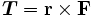# Torque Units Conversion

From
To

Torque Units

The torque units in the torque converter are dyne centimeter, dyne meter, dyne millimeter, gram centimeter, gram meter, gram millimeter, kilogram centimeter, kilogram meter, kilogram millimeter, kilonewton meter, kilopond meter, meganewton meter, micronewton meter, millinewton meter, long tone foot, newton centimeter, newton meter, newton millimeter, ounce foot, ounce inch, pound foot, poundal foot, pound inch, short tone foot.

The most common imperial torque units are the pound foot (lb ft) and pound inch (lb in). The metric torque unit is the newton meter (Nm).

To convert between torque units newton meter and pound foot and for more information, please visit newton meter to pound foot torque converter page.

What is Torque?

Torque is a force that rotates or turns things.

Notice that the torque units contain a distance and a force. To calculate the torque, you multiply the force by the distance from the center. As a formula, you can calculate the torque as:where,

r is the vector from the axis of rotation to the point on which the force is acting.

F is the vector of force.

For example, In the case of the lug nuts, if the wrench is a foot long, and you put 200 pounds of force on it, you are generating 200 pound-feet of torque. If you use a 2-foot wrench, you only need to put 100 pounds of force on it to generate the same torque.

Related Converters
Enter a valid value